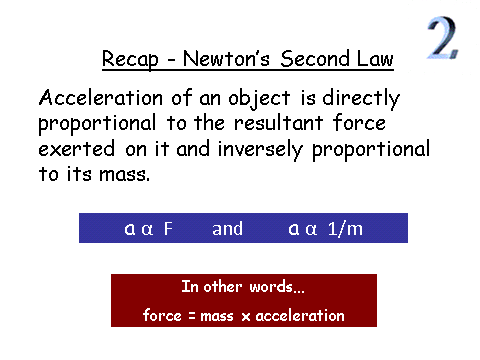# Newton's Second Law

## Newton’s Second Law

Newton’s second law is, perhaps, his most famous law.## F=ma

Force = mass x acceleration

F = ma

Force is measured in Newtons (N)

Mass is measured in kilograms (kg)

Acceleration is measured in metres per second squared (m/s2)

## Inertia

From the equation it is clear to see that if we increase the mass, we have to increase the force applied in order to maintain a constant acceleration. This was mentioned in the previous learning episode. Inertia is a measure of how difficult it is to change the velocity of an object. In order to change the velocity you need to change the acceleration and in order for that to occur, a change in force. The mass in the equation F = ma is sometimes referred to as inertial mass for this reason.

## Approximate

A small point in the grand scheme of the course but you need to know that mathematical symbol for approximately.

Here it is:A bus that accelerates at a rate of 0.5m/s2 when 5 people push it, each with a force of 750N, what is the mass of the bus?